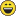# Format lists and arrays

1710
3
09-01-2016 07:08 AM
Labels (1)byMVP Esteemed Contributor
1 3 1,710

I have been working with arrays and lists a lot lately and I wanted to view them in column mode rather than in row mode to make documentation easier to follow and to make the output more intuitative.  The demo relies on the numpy module, but that is no issue since everyone has it with their ArcMap and ArcGIS Pro installation.

You can alter where an array gets parsed by changing the values in this line...

np.set_printoptions(edgeitems=2,linewidth=80,precision=2,suppress=True,threshold=8)

I altered the [ and ] characters common in lists and arrays, just to show you could do it.  I also added an indentation option.

If you like the array output better than the list output, you can simply make an array from a list using ... new_array np.array(input_list) ...

``"""Script:    array_print_demo.pyAuthor:    Dan.Patterson@carleton.caModified:  2016-01-16Purpose:   Demonstration of formatting arrays/lists in alternate formatsFunctions:  -  frmt_arr(arr, indents=0)  -  indent_arr(arr, indents=1)  called by above"""import numpy as npnp.set_printoptions(edgeitems=2,linewidth=80,precision=2,suppress=True,threshold=8)def frmt_arr(arr, indents=0):    """     Format arrays or lists with dimensions >=3 printed by rows rather    than the usual column expression.  Indentation can be employed as well as    the number of indent levels.  See string.expandtabs to alter the tab level.    """    if isinstance(arr, list):        arr = np.asarray(arr)  # make sure inputs are array    result=[]; temp = []    arr_dim = arr.ndim    if arr_dim < 3:        if (arr_dim == 1) and (len(arr) > 1):            arr = arr.reshape((arr.shape[-1],1))        if indents:            arr = indent_arr(arr, indents)        return arr    elif arr_dim == 3:        temp.append(arr)    elif arr_dim > 3:        temp = [sub for sub in arr]        for arr in temp:         for x in zip(*arr):            result.append("   ".join(map(str,x))) # use tabs \t, not space        if arr_dim > 3:            result.append("----")        out = "\n".join(result)    if indents:        out = indent_arr(out, indents)        #out = (str(out).replace("[","|")).replace("]","|")        #tabs = "    "*indents # "\t"        # out = tabs + out.replace("\n","\n" + tabs)    return outdef indent_arr(arr, indents=1):    """    Add an indent to a str or repr version of an array.    The number of indents is determined by the indents option.    """            out = (str(arr).replace("[","|")).replace("]","|")    tabs = "    "*indents # "\t"    out = tabs + out.replace("\n","\n" + tabs)    return outif __name__=='__main__':    """largely a demonstration of slicing by array dimensions    """    # syntax  frmt_arr(arr, indents=1)    a = np.random.randint(1,1000,16)    a1 = a.tolist()    e = a.reshape((4,2,2)); e1 = d.tolist()‍‍‍‍‍‍‍‍‍‍‍‍‍‍‍‍‍‍‍‍‍‍‍‍‍‍‍‍‍‍‍‍‍‍‍‍‍‍‍‍‍‍‍‍‍‍‍‍‍‍‍‍‍‍‍‍‍‍‍‍‍‍``

Your preference

``>>> print(a1)[[135, 944], [196, 335], [761, 521], [529, 687], [803, 393], [254, 797], [610, 605], [328, 516]] >>> # or ...>>> print(frmt_arr(a1,indents=1))    ||135 944|     |196 335|     ...,      |610 605|     |328 516||A 3D list or array>>> print(e)[[[135 944]  [196 335]] [[761 521]  [529 687]] [[803 393]  [254 797]] [[610 605]  [328 516]]]>>> # or ...>>> print(frmt_arr(e,indents =2))        |135 944|   |761 521|   |803 393|   |610 605|        |196 335|   |529 687|   |254 797|   |328 516|‍‍‍‍‍‍‍‍‍‍‍‍‍‍‍‍‍‍‍‍‍‍‍‍``
Tags (5)
3 CommentsbyEsri Esteemed Contributor

Cool! Thanx for sharing Dan!byMVP Frequent Contributor

pandas version:

import numpy

from pandas import *

a1 = [[135, 944], [196, 335], [761, 521], [529, 687], [803, 393], [254, 797], [610, 605], [328, 516]]

df = pandas.DataFrame(a1)

print df.values

result:

 [[135 944] [196 335] [761 521] [529 687] [803 393] [254 797] [610 605] [328 516]]byMVP Esteemed Contributor

but is lines 23 and 24 that are the cool linesAbout the Author
Retired Geomatics Instructor at Carleton University. I am a forum MVP and Moderator. Current interests focus on python-based integration in GIS. See... Py... blog, my GeoNet blog...
Labels